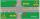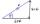# Pythagorean theorem + fundamentals of Physics - examples

1. ForcesIn point O acts three orthogonal forces: F1 = 20 N, F2 = 7 N and F3 = 19 N. Determine the resultant of F and the angles between F and forces F1, F2 and F3.
2. Widescreen monitorComputer business hit by a wave of widescreen monitors and televisions. Calculate the area of ​​the LCD monitor with a diagonal size 20 inches at ratio 4:3 and then 16:9 aspect ratio. Is buying widescreen monitors with same diagonal more advantageous tha
3. Two forcesTwo forces with magnitudes of 25 and 30 pounds act on an object at angles of 10° and 100° respectively. Find the direction and magnitude of the resultant force. Round to two decimal places in all intermediate steps and in your final answer.
4. JourneyCharles and Eva stands in front of his house, Charles went to school south at speed 5.4 km/h, Eva went to the store on a bicycle eastwards at speed 21.6 km/h. How far apart they are after 10 minutes?
5. Acceleration 2if a car traveling at a velocity of 80 m/s/south accelerated to a velocity of 100 m/s east in 5 seconds, what is the cars acceleration? using Pythagorean theorem
6. ForcesDetermine the resultant of two perpendicular forces F1 = 560 N and second force of 25% smaller.
7. Two aircraftFrom the airport will start simultaneously two planes, which fly tracks are perpendicular to each other. The first flying speed of 680 km/h and the second 840 km/h. Calculate how far the aircraft will fly for half an hour.
8. Forces on earth directionsA force of 60 N [North] and 80 N [East] is exerted on an object wigth 10 kg. What is the acceleration of the object?
9. The swimmerThe swimmer swims at a constant speed of 0.85 m/s relative to water flow. The current speed in the river is 0.40 m/s, the river width is 90 m. a) What is the resulting speed of the swimmer with respect to the tree on the riverbank when the swimmer motion
10. ParatrooperAfter the parachute is opened, the paratrooper drops to the ground at a constant speed of 2 m/s, with the sidewinding at a steady speed of 1.5 m/s. Find: a) the magnitude of its resulting velocity with respect to the ground, b) the distance of his land fr

We apologize, but in this category are not a lot of examples.
Do you have an interesting mathematical example that you can't solve it? Enter it, and we can try to solve it.

To this e-mail address, we will reply solution; solved examples are also published here. Please enter e-mail correctly and check whether you don't have a full mailbox.

Pythagorean theorem is the base for the right triangle calculator.